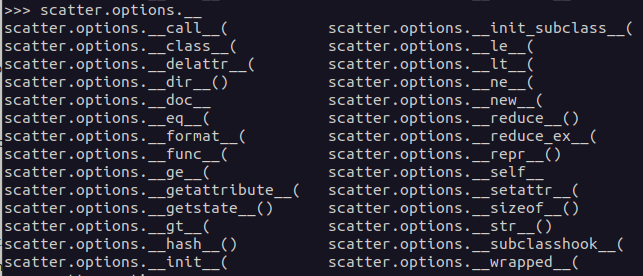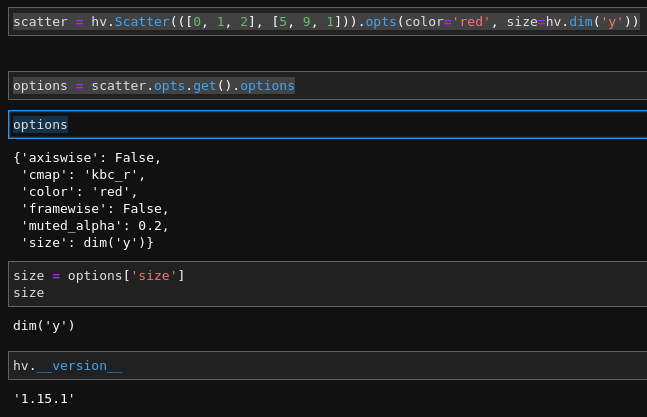# Can I read what opts have been applied to my plot?

Hi, I’ve come across this situation twice, where I want to know what options have been passed to an element’s opts.

Suppose I have the following:

scatter = hv.Scatter(df, ‘x’, ‘y’).opts(color=‘red’)

How can I know the value passed to the color option?

Another, more complex use case is if I have some style mapping based on one column:

scatter = hv.Scatter(df, ‘x’, ‘y’).opts(size=hv.dim(‘y’))

and elsewhere I would like to be able to retrieve the result of the operation applied to the ‘y’ dimension without having to compute it again.

Does anyone know if that is possible?

1 Like

Hi @Theom,

I think maybe this

``````scatter.opts.info()
``````

Thanks @carl. For me (with holoviews 1.15.1) this does print out the options in Jupyter or a console but returns None.

Hi @Theom2,

So looks like info is to display but I think you can do something like

``````colour = scatter.options.get(‘color’)
``````

Should return a string here

try typing scatter.options. now hit tab should show you some handy stuff

Hope it helps, Carl.

Thanks again @carl.

I get an error though. There is no `get` method for me, neither with holoviews 1.15.1 nor 1.15.4.

Plus trying to see where I can go from `.options` does not yield much that is interesting:Try this (holoviews 1.15.1):

``````params = scatter.opts.get()
params.options['color']
``````

This returns `'red'`

2 Likes

With respect to that one:

This here works, but don’t ask me why.

``````params = scatter.opts.get()
params['size']
``````

returns the dim transform object:
`dim('y')`

2 Likes

Thanks @Jan, it works for me!

Actually I can also get the size option with `params.options['size']`.

NB: What you get is not the computed result on the y dimension (i.e. not `hv.dim('y').apply(dataset)`). But that’s already useful.

2 Likes

Strange. I get:

`The options property may only be used with non-cyclic Options.`

Not sure if you now have a newer version than 1.15.1 and that’s why it works for you?

Strange indeed! For me it works both with 1.15.1 and 1.15.4.Okay. that works for me too. But here comes the crazy part:

If I do this, it works.

``````scatter = hv.Scatter(df1, 'x', 'y').opts(color='red',
size=hv.dim('y'))
params = scatter.opts.get()
params.options['size']    # output: dim('y')
``````

If I remove the `color='red'`, it doesn’t work:

``````scatter = hv.Scatter(df1, 'x', 'y').opts(size=hv.dim('y'))
params = scatter.opts.get()
params.options['size']    # error
``````

Looks like a bug to me.

1 Like